## Monday, August 28, 2017

### Adaptive Strawberry Algorithm?

In (1) a so-called Adaptive Plant Propagation or Adaptive Strawberry Algorithm is applied to a standard Economic Load Dispatch problem.

I had never heard of adaptive strawberries or about this dispatch problem being difficult enough to warrant a heuristic approach.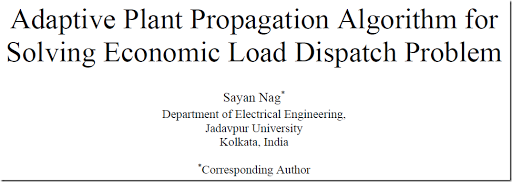##### Abstract

Optimization problems in design engineering are complex by nature, often because of the involvement of critical objective functions accompanied by a number of rigid constraints associated with the products involved. One such problem is Economic Load Dispatch (ED) problem which focuses on the optimization of the fuel cost while satisfying some system constraints. Classical optimization algorithms are not sufficient and also inefficient for the ED problem involving highly nonlinear, and non-convex functions both in the objective and in the constraints. This led to the development of metaheuristic optimization approaches which can solve the ED problem almost efficiently. This paper presents a novel robust plant intelligence based Adaptive Plant Propagation Algorithm (APPA) which is used to solve the classical ED problem. The application of the proposed method to the 3-generator and 6-generator systems shows the efficiency and robustness of the proposed algorithm. A comparative study with another state-of-the-art algorithm (APSO) demonstrates the quality of the solution achieved by the proposed method along with the convergence characteristics of the proposed approach.

##### Problem

The economic dispatch problem used in this paper can be stated as a simple convex QP (Quadratic Programming) problem:

\bbox[lightcyan,10px,border:3px solid darkblue]{ \begin{align}\min\>&z = \sum_i (a_i + b_i x_i + c_i x_i^2)\\ & \sum_i x_i = D\\ & \ell_i \le x_i \le u_i\end{align} }

Basically with have $$n$$ generators that have a quadratic cost function w.r.t. to their output $$x_i$$. The constraints deal with meeting demand and operating limits.

Nitpick: the paper mentions: $$\ell_i < x_i < u_i$$ which is mathematically not really ok.

Opposed to what the abstract is saying this is not a non-convex problem, and standard QP algorithms will be able to solve this problem without any problem. There really is no good reason for using a heuristic for this problem.

##### Example 1

The first example is a tiny problem with three generators.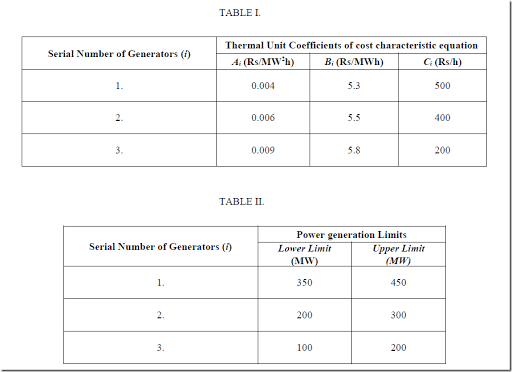Note that the columns in table 1 are mislabeled. The first column represents $$c_i$$ while the last column is $$a_i$$.

The solution is reported as: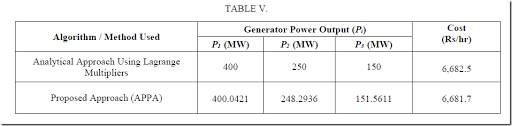The solution calculated by hand seems correct (I guess the author was lucky here that no operating limits were binding). The heuristic gives a solution that is slightly better (lower cost) but that is because this solution is slightly infeasible: we don’t quite match the demand of 800 MW.

##### Example 2

This is another tiny problem with six generators.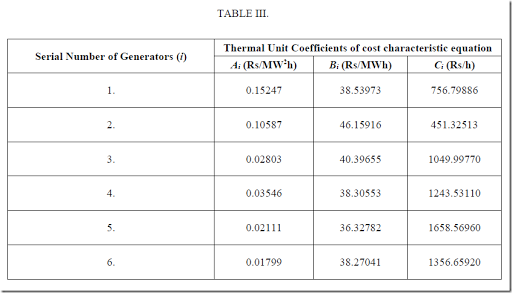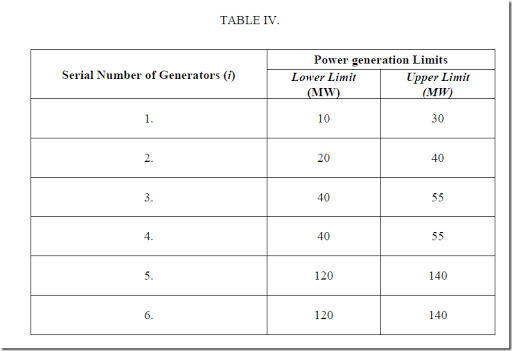The demand is 410 MW. The author shows two solutions: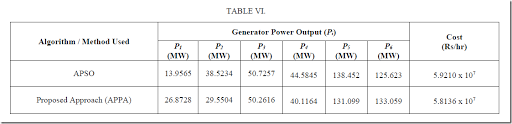These solutions are both wrong. The reported objective values (Cost per hour) should be an indication something is not right. The reason is that the author is confused about the (mislabeling of the) coefficients $$a_i$$, $$b_i$$, $$c_i$$ in table III. The large coefficients $$C_i$$ deal with the fixed cost while the small coefficients $$A_i$$ are for the quadratic terms.

The correct solution is: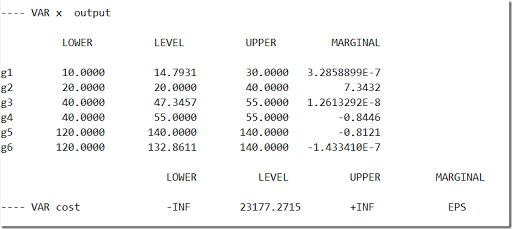A QP solver can solve this model to (proven) optimality in less than a second (Cplex takes 0.09 seconds).

##### Unit Commitment Problem

A more interesting problem is where we can turn generating units on and off. This is called the unit-commitment problem. Often exotic things like ramp-up and ramp-down limits, and other operational constraints, are added. These problems can actually become very difficult to solve, and heuristics pay traditionally an important role here. Having said that, there is somewhat of an increased interest to solve these models with MIP technology (after piecewise linear representation of the quadratic costs) due to substantial advances in state-of-the-art solvers. I have not seen many efforts to use MIQP algorithms here: often these linearized versions work quite well.

##### Conclusion

Writing a solid paper is not that easy.

##### References
1. Sayan Nag, Adaptive Plant Propagation Algorithm for Solving Economic Load Dispatch Problem, 2017 [link]